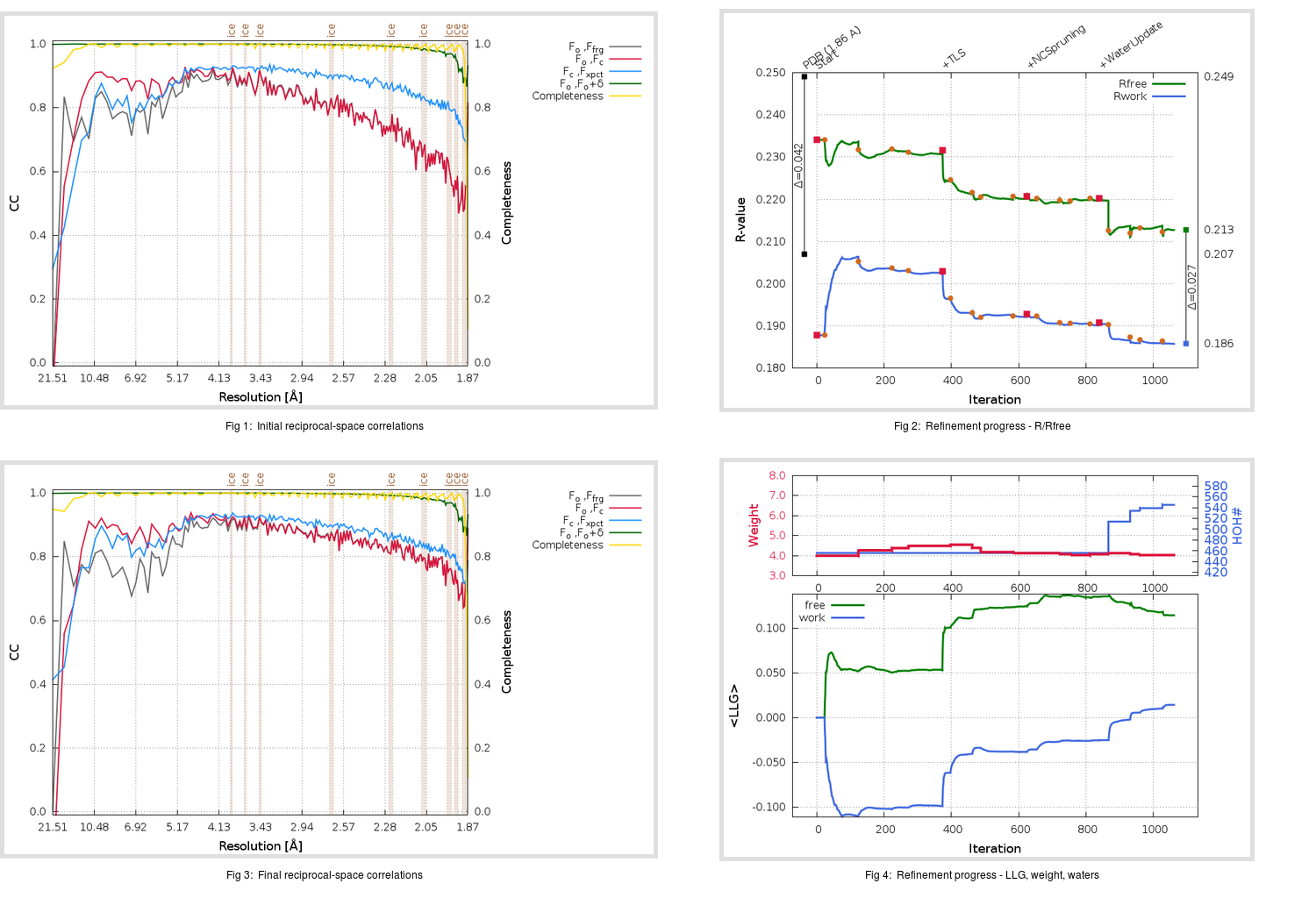Content:

```    Diffraction limits & principal axes of ellipsoid fitted to diffraction cut-off surface:
1.825         0.9985   0.0000  -0.0553       0.941 a* - 0.338 c*
1.852         0.0000   1.0000   0.0000       b*
1.835         0.0553   0.0000   0.9985       0.044 a* + 0.999 c*
```

## Deposited

` `
 Date deposited Date data collection Resolution R, Rfree 20030726 20030718 1.86 0.1940 0.2490

Molprobity (CCP4 7.0 version) summary:

```Ramachandran outliers =   0.00 %
favored =  97.43 %
Rotamer outliers      =   5.00 %
C-beta deviations     =     3
Clashscore            =   7.36
RMS(bonds)            =   0.0184
RMS(angles)           =   1.73
MolProbity score      =   2.05
Resolution            =   1.86
R-work                =   0.1940
R-free                =   0.2490
```

```Number of waters      =   456

<B> (all atoms) =   30.98 ( sd =    9.76 ) for       5104 non-hydrogen atoms
<B>   (protein) =   30.04 ( sd =    9.25 ) for       4640 non-hydrogen atoms
<B>     (water) =   40.40 ( sd =    9.68 ) for        456 non-hydrogen atoms
<B>    (others) =   28.45 ( sd =    1.16 ) for          8 non-hydrogen atoms

B min/max       (all non-hydrogen atoms) =   13.04 /   74.77
B min/max   (protein non-hydrogen atoms) =   13.04 /   66.10
B min/max     (water non-hydrogen atoms) =   17.68 /   74.77
B min/max     (other non-hydrogen atoms) =   27.28 /   30.34
```

## BUSTER (re-)refinement

` `

Molprobity (CCP4 7.0 version) summary:

```Ramachandran outliers =   0.00 %
favored =  98.12 %
Rotamer outliers      =   2.50 %
C-beta deviations     =     0
Clashscore            =   4.33
RMS(bonds)            =   0.0121
RMS(angles)           =   1.52
MolProbity score      =   1.52
Resolution            =   1.86
R-work                =   0.1858
R-free                =   0.2128
```

```Number of waters      =   545

<B> (all atoms) =   33.04 ( sd =   11.12 ) for       5193 non-hydrogen atoms
<B>   (protein) =   31.78 ( sd =   10.35 ) for       4640 non-hydrogen atoms
<B>     (water) =   43.71 ( sd =   11.50 ) for        545 non-hydrogen atoms
<B>    (others) =   27.12 ( sd =    0.20 ) for          8 non-hydrogen atoms

B min/max       (all non-hydrogen atoms) =   14.82 /   92.93
B min/max   (protein non-hydrogen atoms) =   14.82 /   74.01
B min/max     (water non-hydrogen atoms) =   18.92 /   92.93
B min/max     (other non-hydrogen atoms) =   26.78 /   27.43
```

Refinement progression:Results:

` `
 File Remark 1Q2W_aB_refine.01_04_refine.pdb.gz exact refinement commands are in header 1Q2W_aB_refine.01_04_refine.mtz.gz including original deposited data and several re-refinement map coefficients 1Q2W_aB_refine.01_04_BUSTER_model.cif.gz including any non-standard compound restraints 1Q2W_aB_refine.01_04_BUSTER_refln.cif.gz